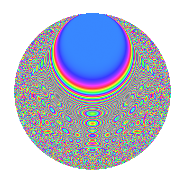# Properties

 Label 8042.2.aLevel 8042 Weight 2 Character orbit a Rep. character $$\chi_{8042}(1,\cdot)$$ Character field $$\Q$$ Dimension 336 Newforms 4 Sturm bound 2011 Trace bound 1

# Related objects

## Defining parameters

 Level: $$N$$ = $$8042 = 2 \cdot 4021$$ Weight: $$k$$ = $$2$$ Character orbit: $$[\chi]$$ = 8042.a (trivial) Character field: $$\Q$$ Newforms: $$4$$ Sturm bound: $$2011$$ Trace bound: $$1$$

## Dimensions

The following table gives the dimensions of various subspaces of $$M_{2}(\Gamma_0(8042))$$.

Total New Old
Modular forms 1007 336 671
Cusp forms 1004 336 668
Eisenstein series 3 0 3

The following table gives the dimensions of the cuspidal new subspaces with specified eigenvalues for the Atkin-Lehner operators and the Fricke involution.

$$2$$$$4021$$FrickeDim.
$$+$$$$+$$$$+$$$$82$$
$$+$$$$-$$$$-$$$$86$$
$$-$$$$+$$$$-$$$$101$$
$$-$$$$-$$$$+$$$$67$$
Plus space$$+$$$$149$$
Minus space$$-$$$$187$$

## Trace form

 $$336q$$ $$\mathstrut -\mathstrut 2q^{3}$$ $$\mathstrut +\mathstrut 336q^{4}$$ $$\mathstrut -\mathstrut 2q^{5}$$ $$\mathstrut +\mathstrut 334q^{9}$$ $$\mathstrut +\mathstrut O(q^{10})$$ $$336q$$ $$\mathstrut -\mathstrut 2q^{3}$$ $$\mathstrut +\mathstrut 336q^{4}$$ $$\mathstrut -\mathstrut 2q^{5}$$ $$\mathstrut +\mathstrut 334q^{9}$$ $$\mathstrut -\mathstrut 12q^{11}$$ $$\mathstrut -\mathstrut 2q^{12}$$ $$\mathstrut +\mathstrut 10q^{13}$$ $$\mathstrut +\mathstrut 4q^{14}$$ $$\mathstrut +\mathstrut 4q^{15}$$ $$\mathstrut +\mathstrut 336q^{16}$$ $$\mathstrut +\mathstrut 8q^{17}$$ $$\mathstrut +\mathstrut 8q^{18}$$ $$\mathstrut +\mathstrut 8q^{19}$$ $$\mathstrut -\mathstrut 2q^{20}$$ $$\mathstrut +\mathstrut 20q^{21}$$ $$\mathstrut -\mathstrut 6q^{22}$$ $$\mathstrut +\mathstrut 4q^{23}$$ $$\mathstrut +\mathstrut 330q^{25}$$ $$\mathstrut +\mathstrut 4q^{26}$$ $$\mathstrut +\mathstrut 4q^{27}$$ $$\mathstrut -\mathstrut 12q^{30}$$ $$\mathstrut -\mathstrut 12q^{31}$$ $$\mathstrut +\mathstrut 12q^{33}$$ $$\mathstrut -\mathstrut 8q^{34}$$ $$\mathstrut -\mathstrut 4q^{35}$$ $$\mathstrut +\mathstrut 334q^{36}$$ $$\mathstrut +\mathstrut 2q^{37}$$ $$\mathstrut -\mathstrut 2q^{38}$$ $$\mathstrut +\mathstrut 16q^{39}$$ $$\mathstrut -\mathstrut 4q^{41}$$ $$\mathstrut -\mathstrut 8q^{42}$$ $$\mathstrut -\mathstrut 12q^{43}$$ $$\mathstrut -\mathstrut 12q^{44}$$ $$\mathstrut -\mathstrut 6q^{45}$$ $$\mathstrut +\mathstrut 4q^{46}$$ $$\mathstrut +\mathstrut 24q^{47}$$ $$\mathstrut -\mathstrut 2q^{48}$$ $$\mathstrut +\mathstrut 340q^{49}$$ $$\mathstrut +\mathstrut 4q^{51}$$ $$\mathstrut +\mathstrut 10q^{52}$$ $$\mathstrut +\mathstrut 14q^{53}$$ $$\mathstrut -\mathstrut 4q^{55}$$ $$\mathstrut +\mathstrut 4q^{56}$$ $$\mathstrut +\mathstrut 28q^{57}$$ $$\mathstrut -\mathstrut 2q^{58}$$ $$\mathstrut -\mathstrut 46q^{59}$$ $$\mathstrut +\mathstrut 4q^{60}$$ $$\mathstrut +\mathstrut 30q^{61}$$ $$\mathstrut +\mathstrut 20q^{62}$$ $$\mathstrut +\mathstrut 48q^{63}$$ $$\mathstrut +\mathstrut 336q^{64}$$ $$\mathstrut +\mathstrut 16q^{65}$$ $$\mathstrut +\mathstrut 22q^{67}$$ $$\mathstrut +\mathstrut 8q^{68}$$ $$\mathstrut +\mathstrut 20q^{69}$$ $$\mathstrut -\mathstrut 4q^{70}$$ $$\mathstrut +\mathstrut 8q^{71}$$ $$\mathstrut +\mathstrut 8q^{72}$$ $$\mathstrut +\mathstrut 24q^{74}$$ $$\mathstrut +\mathstrut 18q^{75}$$ $$\mathstrut +\mathstrut 8q^{76}$$ $$\mathstrut -\mathstrut 16q^{77}$$ $$\mathstrut +\mathstrut 4q^{78}$$ $$\mathstrut -\mathstrut 8q^{79}$$ $$\mathstrut -\mathstrut 2q^{80}$$ $$\mathstrut +\mathstrut 320q^{81}$$ $$\mathstrut +\mathstrut 12q^{82}$$ $$\mathstrut +\mathstrut 2q^{83}$$ $$\mathstrut +\mathstrut 20q^{84}$$ $$\mathstrut -\mathstrut 20q^{85}$$ $$\mathstrut +\mathstrut 30q^{86}$$ $$\mathstrut -\mathstrut 12q^{87}$$ $$\mathstrut -\mathstrut 6q^{88}$$ $$\mathstrut +\mathstrut 16q^{89}$$ $$\mathstrut +\mathstrut 4q^{90}$$ $$\mathstrut +\mathstrut 36q^{91}$$ $$\mathstrut +\mathstrut 4q^{92}$$ $$\mathstrut +\mathstrut 20q^{93}$$ $$\mathstrut -\mathstrut 12q^{94}$$ $$\mathstrut -\mathstrut 20q^{95}$$ $$\mathstrut -\mathstrut 12q^{97}$$ $$\mathstrut +\mathstrut 40q^{98}$$ $$\mathstrut -\mathstrut 132q^{99}$$ $$\mathstrut +\mathstrut O(q^{100})$$

## Decomposition of $$S_{2}^{\mathrm{new}}(\Gamma_0(8042))$$ into irreducible Hecke orbits

Label Dim. $$A$$ Field CM Traces A-L signs $q$-expansion
$$a_2$$ $$a_3$$ $$a_5$$ $$a_7$$ 2 4021
8042.2.a.a $$67$$ $$64.216$$ None $$67$$ $$-11$$ $$-20$$ $$-40$$ $$-$$ $$-$$
8042.2.a.b $$82$$ $$64.216$$ None $$-82$$ $$-13$$ $$3$$ $$-37$$ $$+$$ $$+$$
8042.2.a.c $$86$$ $$64.216$$ None $$-86$$ $$12$$ $$-4$$ $$35$$ $$+$$ $$-$$
8042.2.a.d $$101$$ $$64.216$$ None $$101$$ $$10$$ $$19$$ $$42$$ $$-$$ $$+$$

## Decomposition of $$S_{2}^{\mathrm{old}}(\Gamma_0(8042))$$ into lower level spaces

$$S_{2}^{\mathrm{old}}(\Gamma_0(8042)) \cong$$ $$S_{2}^{\mathrm{new}}(\Gamma_0(4021))$$$$^{\oplus 2}$$